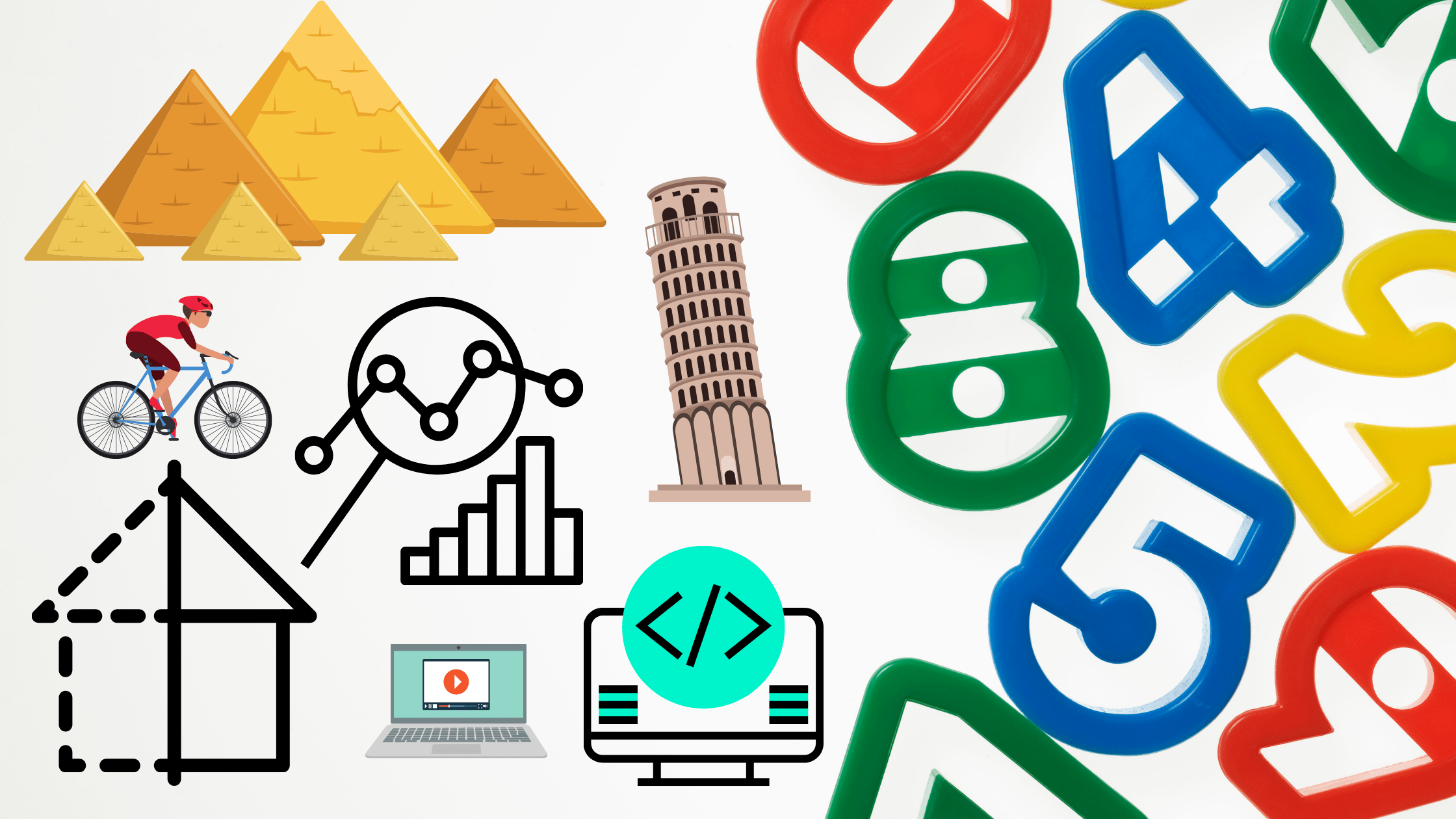# Ontario De-Streamed Grade 9 Math Curriculum Explained (+ Resources)School is just around the corner and grade 9 students in Ontario, Canada who are taking the de-streamed grade 9 math course (MTH1W) will be learning based on the new grade 9 math curriculum.

In this blog post we will go over the components of the new Ontario grade 9 math curriculum and offer some helpful grade 9 math resources.

So, the new curriculum consists of the following strands:

AA. Social-Emotional Learning (SEL) Skills in Mathematics

A. Mathematical Thinking and Making Connections

B. Number

C. Algebra

D. Data

E. Geometry and Measurement

F. Financial Literacy

## Ontario Grade 9 Math Curriculum Strands Overview

The SEL skills in mathematics will be developed through activities and various instructional approaches as students progress through strands B-F.

Strand A is listed separately, but is not really an independent strand. Rather, the expectation is that it will be embedded into Strands B through F of the new grade 9 math curriculum to ensure students have thorough understanding of and fluency with mathematical processes, as well as are able to make connections between the math they learn in class and various real life situations.

Strand B is concerned with understanding number systems and learning about the origins of those systems, based on the discoveries in different cultures; performing math operations on different types of numbers (integers, fractions, decimals), understanding ratios, proportions, percent and evaluating powers.

Strand C focuses on Algebra and incorporates coding. Students will learn how to convert between different mathematical representations of particular situations (for example, translating a word problem into an algebraic expression). Simplifying algebraic expressions and solving equations are also part of this strand of the new grade 9 math curriculum. Strand C also covers linear relations and their applications. In addition, teachers will be asking students to expand their understanding of algebra onto programming by defining variables, setting parameters and writing code to create math tools (a basic calculator in Python, for example).

Keep reading to get to the list of helpful math resources divided by strand

Strand D will provide students with lots of opportunities to connect what they observe and experience in real life with math they learn in class and extend their understanding. Students will be collecting data, analyzing and displaying it. They will learn how to use quartiles and box plots to analyze one variable data and how a scatterplot can help display two variable data. Students will discuss the process of mathematical modelling, as well as try to determine ways to display and analyze real life data in order to create a mathematical model. The strand also gives students an opportunity to make predictions, discuss potential limitations, and present their innovative ideas.

Strand E is all about geometric shapes, measurement and their applications. Students are expected to research and discuss the origin and meaning of geometric shapes in various cultures and designs. Students will work with different measurement systems. They will work on finding area and perimeter, volume and surface area of various 2D and 3D shapes, including composite shapes.

Finally, strand F is dedicated to financial literacy. Students will learn how to make informed financial decisions based on analyzing situations. They will learn about appreciation and depreciation, interest rates and down payments. Students will also analyze sample budgets and create their own.

Strand AA Resources:

30 Powerful Math Quotes

Developing and Fostering Grit in Students

Strand A Resources:

Things you can do to think like Einstein

15 Math Phrases Students Should Know

Strand B Resources:

Video Lesson and Printable Practice: Natural Numbers up to 1000

Video Lesson and Printable Practice: Numbers up to 100,000 and their properties

Math Number Patterns and Cool Number Tricks

The importance of brackets in math

Math tricks involving numbers and powers

Fractions and rational expressions (with history)

Video Lesson and Printable Practice: Operations with Integers

Video Lesson and Printable Practice: Exponents and exponent laws

Strand C Resources:

Equations: their properties and origin

Why software developers need to learn math

Video Lesson and Printable Practice: Polynomials and algebraic expressions

Video Lesson and Printable Practice: Solving linear equations

Video Lesson and Printable Practice: Linear Relations

Online Whiteboard Template: Functions and Python

Strand D Resources:

Gapminder Tools

Learning history through math: analyzing data

Online Whiteboard Template: Data structure and binary trees

Strand E Resources:

Survival Math: If you ever get lost in the wild

Sketchometry Interactive Tool Overview

Different ways to find the area of a triangle

Video Lesson and Printable Practice: Surface area and Volume

Strand F Resources:

Video Lesson and Printable Practice: Money operations and tax

Financial Literacy Resources: Making decisions

1

## One thought on “Ontario De-Streamed Grade 9 Math Curriculum Explained (+ Resources)”

1. Pingback: My Homepage